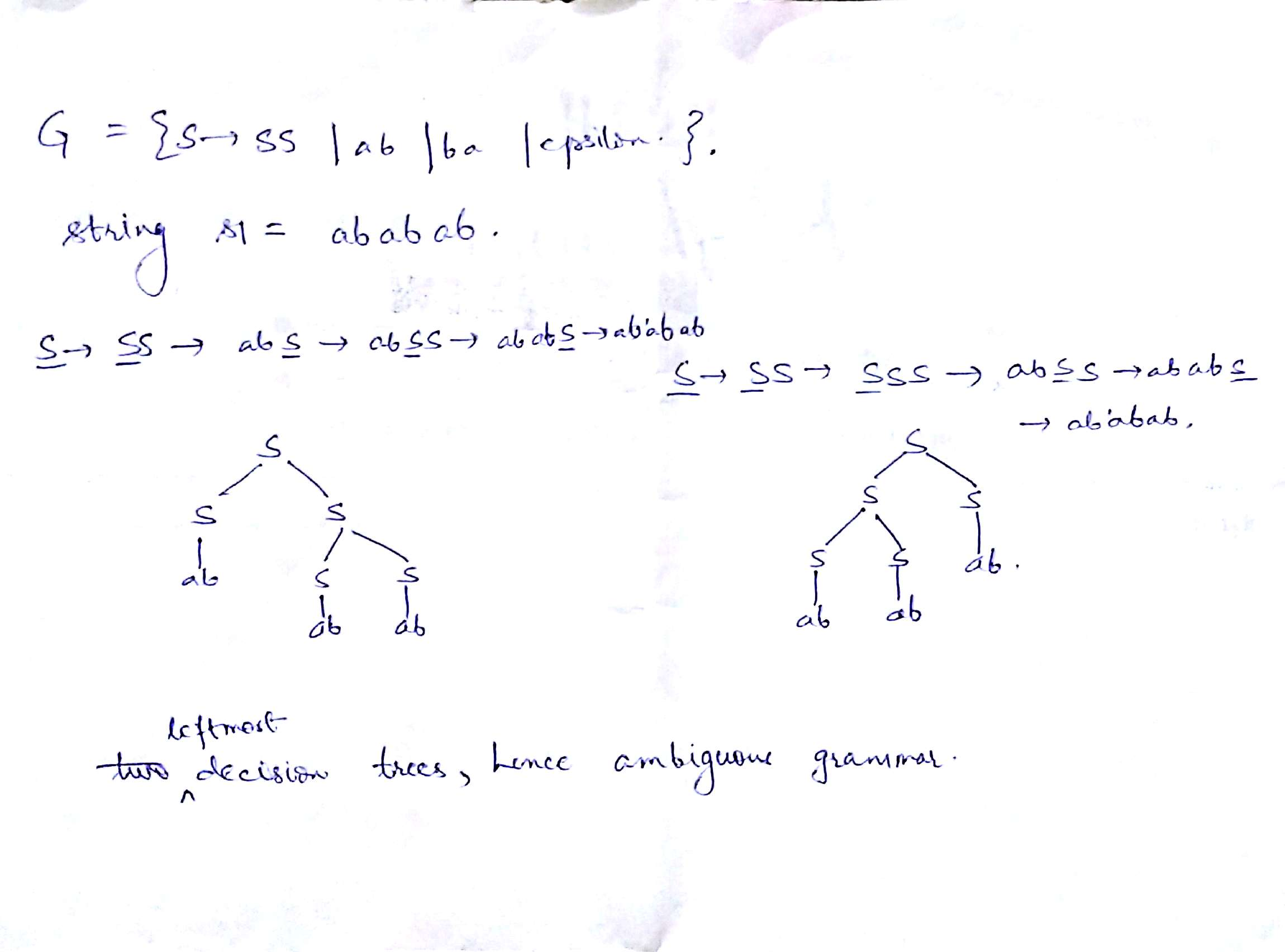# GATE | GATE-CS-2006 | Question 32

• Last Updated : 28 Jun, 2021

Consider the following statements about the context free grammar

```G = {S → SS, S → ab, S → ba, S → Ε}
I. G is ambiguous
II. G produces all strings with equal number of a’s and b’s
III. G can be accepted by a deterministic PDA.```

Which combination below expresses all the true statements about G?
(A) I only
(B) I and III only
(C) II and III only
(D) I, II and III

Explanation:

Statement I: G is ambiguous because, as shown in the image below there can be two decision tree for string S = ababab [TRUE]Statement II: G produces all strings with equal number of a’s and b’s [FALSE]

string ‘aabb’ cannot be produced by G

Statement III: G can be accepted by a deterministic PDA [TRUE]

Assume there is a PDA which pushes if top of the stack is \$ (bottom most alphabet of the stack) and pops otherwise. A string is rejected while popping if the current letter and top of the stack are same. This PDA can derive G.

Hence, correct answer should be (B) I and III only

This solution is contributed by Vineet Purswani.

Quiz of this Question

My Personal Notes arrow_drop_up